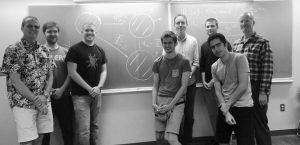# seminar-spring2019

Analysis Seminar Spring 2019Upcoming Seminars (7th hour Wednesdays in LIT368)

• Friday 29 March.
• Speaker: Alan Sola, Stockholm University.
• Title: One-dimensional scaling limits in a Laplacian random growth model
• Abstract: In joint work with Amanda Turner (Lancaster, UK) and Fredrik Viklund (KTH, Sweden) we consider a one-parameter conformal random growth model in which strong feedback in the growth rule leads to degenerate scaling limits. More precisely, we show that there is a phase transition in the parameter that is detected in the ancestral structure of the growing clusters.

 Seminars past Wednesday 13 March. Speaker. James Pascoe. Title: Computing matrix algebra dimension and other exotic applications of the Pick matrix. Abstract: We give an efficient solution to the following problem: Given X_1, . . . , X_d and Y some n by n matrices can we determine if Y is in the unital algebra they generate as a subalgebra of all n by n matrices? The solution also gives an easy method for computing the dimension this algebra. The solution relies on the computation of a certain Pick matrix which arises naturally in the theory of bounded interpolation. We will discuss some related results on noncommutative Pick interpolation, and a technically interesting negative resolution of a conjecture about multipliers on the Fock space. Parts of this talk will concern work with various coauthors, including O’Meara, (refinements and implementation of the algorithm) and Augat, Jury, and Klep (failure of the column-row property on the Fock space.) Wednesday 27 February. Speaker: Douglas Pfeffer Title: Widom Theorem for Finite-Codimensional Subalgeabras of a Class of Uniform Algebras Abstract: Given a function $$\phi \in L^\infty(\mathbb{T}),$$ its Toeplitz operator $$T_\phi$$ is given by $$T_\phi = PM_\phi,$$ where $$P$$ is the orthogonal projection from $$L^2$$ onto $$H^2.$$ In 1960, Harold Widom showed that that $$T_\phi$$ is left-invertible if and only if the distance from $$\phi$$ to $$H^\infty$$ is strictly less than one. Since then, instead of the algebra $$H^\infty,$$ versions of this theorem have been established for the Neil Algebra (2017) and then for the 2-Point Algebra (2018). In this talk, we establish a version of this theorem that holds for all finite-codimensional subalgebras of a broad class of uniform algebras. This work intimately extends the work on both the Neil and 2-Point Algebras, as well as Abrahamse’s 1974 work on establishing a Widom theorem for multiply connected domains. Wednesday 20 February. Speaker: Mike Jury. Title: Unbounded multipliers II. Wednesday 13 February. Speaker: Igor Klep (University of Ljubljana). Title: The Bergman centralizer theorem. Abstract:In this expository talk we present a proof of the Bergman centralizer theorem (the centralizer of a nonconstant noncommutative polynomial is isomorphic to a univariate polynomial ring) using Kontsevich’s quantization, largely following Kanel-Belov et al. Wednesday 6 February. Speaker: Mike Jury. Title: Unbounded multipliers. Wednesday 30 January Speaker: Mark Mancuso (Washington University in St. Louis) Title: Shift Form Construction and Applications Abstract: We discuss a construction that yields a type of compactness result for the closed unit ball of operator tuples in SOT. Applications will be given to strongly continuous free functions on operator domains and convexity. Wednesday 23 January Speaker: Scott McCullough Title: Free rational plurisubharmonic functions. Abstract: Free rational plurisubharmonic functions are characterized. They have a particularly simple form that has a canonical free convexity interpretation.